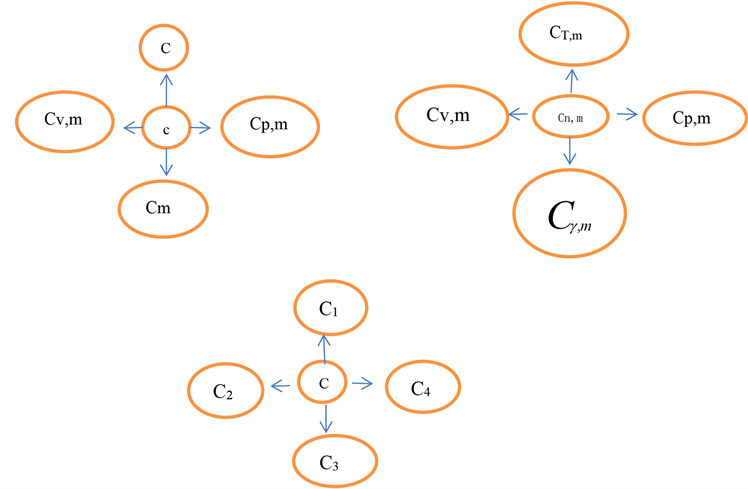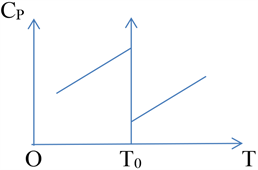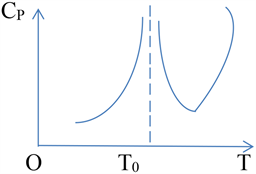#### 期刊菜单

The Analysis How to Guide Students to Learn the Heat Capacity in Thermology Using a Variety of Ways of Thinking
DOI: 10.12677/CES.2021.95194, PDF, HTML, XML, 下载: 44  浏览: 92  科研立项经费支持

Abstract: If the heat capacity is understood better and more deeply, student can analyze correctly the rele-vant problems of heat capacity using a variety of ways of thinking to guide students to understand heat capacity combining with the puzzlement of knowledge of the heat capacity in Thermology. The students’ corresponding thinking ability is cultivated.

1. 引言

2. 引导学生变换思维学习热容知识

2.1. 引导学生由浅入深学习理解热容知识

2.1.1. 热容定义

2.1.2. 比热容性质

2.1.3. 同物质固体与液体比热容情况

(1) 使用类比法解释固体比热容

(2) 同物质液体比固体的比热容大的原因

2.1.4. 热容与温度关系 

(1) 理想气体热容与温度关系Table 1. The general treatment method of heat capacity of ideal gases

(2) 实际气体热容与温度关系

2.2. 引导学生突破固式思维理解热容

(1) 热容是过程量

(2) 热容可为负值 

(3) 利用等容热容求解系统内能变化适用于任何过程

(4) 建立“一展多”模型2.3. 引导学生利用物理思想与理论推导理解特殊点处物质的热容，助培学生科研思维

(1) 一级相变点处的热容

${\left(\frac{\partial {G}_{1}}{\partial T}\right)}_{P}\ne {\left(\frac{\partial {G}_{2}}{\partial T}\right)}_{P}$${\left(\frac{\partial G}{\partial T}\right)}_{P}=-S$${\left(\frac{{\partial }^{2}G}{\partial {T}^{2}}\right)}_{P}=-{\left(\frac{\partial S}{\partial T}\right)}_{P}=-\frac{1}{T}{\left(\frac{\partial Q}{\partial T}\right)}_{P}=-\frac{{C}_{P}}{T}$Figure 2. The change of the heat capacity along with the temperature in the first-order phaseFigure 3. The change of the heat capacity along with the temperature in the second-order phase

(2) 二级相变点处的热容变化

${G}_{\text{1}}={G}_{\text{2}}$${\left(\frac{\partial {G}_{1}}{\partial T}\right)}_{P}={\left(\frac{\partial {G}_{2}}{\partial T}\right)}_{P}$${\left(\frac{{\partial }^{2}{G}_{1}}{\partial {T}^{2}}\right)}_{P}\ne {\left(\frac{{\partial }^{2}{G}_{2}}{\partial {T}^{2}}\right)}_{P}$

$\because$ ${\left(\frac{\partial G}{\partial T}\right)}_{P}=-S$${\left(\frac{{\partial }^{2}G}{\partial {T}^{2}}\right)}_{P}=-{\left(\frac{\partial S}{\partial T}\right)}_{P}=-\frac{1}{T}{\left(\frac{\partial Q}{\partial T}\right)}_{P}=-\frac{{C}_{P}}{T}$${G}_{\text{1}}={G}_{\text{2}}$${G}_{\text{1}}={G}_{\text{2}}$${C}_{{P}_{1}}\ne {C}_{{P}_{2}}$

(3) 绝对零度处物质的比热容 

3. 结论

2019年教育部高等学校大学物理课程教学研究立项项目(DJZW201934xn)；六盘水师范学院教学内容和课程体系改革研究项目(LPSSYjg-2021-20)；六盘水师范学院一流课程《热学》课程建设项目(LPSSYylkc202105)；六盘水师范学院科技创新团队项目(LPSSYKJTD201901)；国家自然科学基金项目(12065016)；关于混合式教学模式下的大学物理课程教学实践和应用(2018520116)；六盘水师范学院硕士学位培育点学科建设项目(LPSSYSSDYPY201704)；六盘水师范学院教学内容与课程体系改革项目(LPSSYjg201931)。

  刘峰, 张旭. 非平衡凝固与固态相变的一体化研究[J]. 金属学报, 2018, 54(5): 702-715.  蔡绍洪. 平衡与非平衡两类相变的临界联系[J]. 电子科技大学学报, 1997(26): 171-180.  魏秀芳. 金属比热容与温度关系的实验研究[J]. 高教期刊, 2015(5): 53-56.  李椿, 章立源, 钱尚武. 热学[M]. 第二版. 北京: 人民教育出版社, 2010: 168-183.  付秦生. 工程热力学[M]. 北京: 机械工业出版社, 2013: 66-71.  张金锋, 代凯, 等. 基于理想气体多方过程的摩尔热容计算[J]. 廊坊师范学院学报, 2017(17): 51-56.  汪志诚. 热力学•统计物理[M]. 第四版. 北京: 高等教育出版社, 2008: 168-183.  秦允豪. 热学[M]. 第四版. 北京: 高等教育出版社, 2018: 168-183.